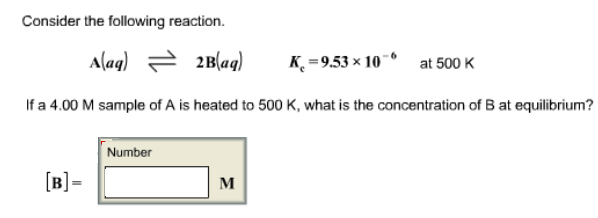# Problem: Consider the following reaction. A (aq) ⇌ 2B (aq)   Kc = 9.53 x 10-6 at 500 K If a 4.00 M sample of A is heated to 500 K, what is the concentration of B at equilibrium?

###### FREE Expert Solution
95% (471 ratings)###### Problem Details

Consider the following reaction.

A (aq) ⇌ 2B (aq)   Kc = 9.53 x 10-6 at 500 K

If a 4.00 M sample of A is heated to 500 K, what is the concentration of B at equilibrium?